# Ellipse

﻿
EllipseAn ellipse obtained as the intersection of a cone with a plane.The rings of Saturn are circular, but when seen partially edge on, as in this photograph, they appear to be ellipses. Photo by ESO

In geometry, an ellipse (from Greek ἔλλειψις elleipsis, a "falling short") is a plane curve that results from the intersection of a cone by a plane in a way that produces a closed curve. Circles are special cases of ellipses, obtained when the cutting plane is orthogonal to the cone's axis. An ellipse is also the locus of all points of the plane whose distances to two fixed points add to the same constant.

Ellipses are closed curves and are the bounded case of the conic sections, the curves that result from the intersection of a circular cone and a plane that does not pass through its apex; the other two (open and unbounded) cases are parabolas and hyperbolas. Ellipses arise from the intersection of a right circular cylinder with a plane that is not parallel to the cylinder's main axis of symmetry. Ellipses also arise as images of a circle under parallel projection and the bounded cases of perspective projection, which are simply intersections of the projective cone with the plane of projection. It is also the simplest Lissajous figure, formed when the horizontal and vertical motions are sinusoids with the same frequency.

## Elements of an ellipseThe ellipse and some of its mathematical properties.

An ellipse is a smooth closed curve which is symmetric about its horizontal and vertical axes. The distance between antipodal points on the ellipse, or pairs of points whose midpoint is at the center of the ellipse, is maximum along the major axis or transverse diameter, and a minimum along the perpendicular minor axis or conjugate diameter.

The semi-major axis (denoted by a in the figure) and the semi-minor axis (denoted by b in the figure) are one half of the major and minor diameters, respectively. These are sometimes called (especially in technical fields) the major and minor semi-axes, the major and minor semiaxes, or major radius and minor radius.

The foci of the ellipse are two special points F1 and F2 on the ellipse's major axis and are equidistant from the center point. The sum of the distances from any point P on the ellipse to those two foci is constant and equal to the major diameter ( PF1 + PF2 = 2a ). Each of these two points is called a focus of the ellipse.

Refer to the lower Directrix section of this article for a second equivalent construction of an ellipse.

The eccentricity of an ellipse, usually denoted by ε or e, is the ratio of the distance between the two foci, to the length of the major axis or e = 2f/2a = f/a. For an ellipse the eccentricity is between 0 and 1 (0<e<1). When the eccentricity is 0 the foci coincide with the center point and the figure is a circle. As the eccentricity tends toward 1, the ellipse gets a more elongated shape. It tends towards a line segment (see below) if the two foci remain a finite distance apart and a parabola if one focus is kept fixed as the other is allowed to move arbitrarily far away.
The distance ae from a focal point to the centre is called the linear eccentricity of the ellipse (f = ae).

## Drawing ellipses

### The pins-and-string method

Video for this method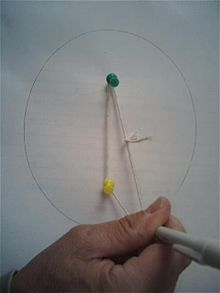Drawing an ellipse with two pins, a loop, and a pen.

An ellipse can be drawn using two drawing pins, a length of string, and a pencil:

Push the pins into the paper at two points, which will become the ellipse's foci. Tie the string into a loose loop around the two pins. Pull the loop taut with the pen's tip, so as to form a triangle. Move the pen around, while keeping the string taut, and its tip will trace out an ellipse. Using two pegs and a rope, this procedure is traditionally used by gardeners to outline an elliptical flower bed; thus it is called the gardener's ellipse.

If the ellipse is to be inscribed within a specified rectangle,[diagram needed] tangent to its four sides at their midpoints, one must first determine the position of the foci and the length of the string loop:

Let A,B,C,D be the corners of the rectangle, in clockwise order, with A-B being one of the long sides. Draw a circle centered on A, whose radius is the short side A-D. From corner B draw a tangent to the circle. The length L of this tangent is the distance between the foci. This length L can be calculated with the Pythagorean theorem. As the tangent is at a right angles to the radius at the intersect of the tangent with the circle L equals square root ((A-B)squared - (A-D)squared) i.e. square root of the long side of the rectangle squared minus short side squared. Draw a horizontal line through the center of the rectangle. This will be the major axis of the ellipse. Place the foci on the major axis, at distance L/2 from the center.

To adjust the length of the string loop, insert a pin at one focus, and the second pin at the opposite side of the rectangle on the major axis. Loop the string around the two pins and tie it taut. Move the second pin to the other focus. Then draw the ellipse as above; it should fit snugly in the original rectangle. Unfortunately strings tend to be elastic so if you push harder on the pencil stretching the string more you will get a bigger ellipse, pushing less it will be smaller. It may take a few tries to push just hard enough to make the ellipse fit the rectangle. The string method was developed by James Clerk Maxwell, the discoverer of the electromagnetic nature of light, at age 12.

### Other methods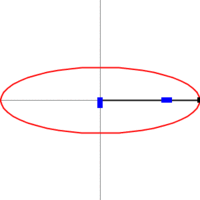Trammel of Archimedes (ellipsograph) animation

An ellipse can also be drawn using a ruler, a set square, and a pencil:

Draw two perpendicular lines M,N on the paper; these will be the major and minor axes of the ellipse. Mark three points A, B, C on the ruler. A->C being the length of the major axis and B->C the length of the minor axis. With one hand, move the ruler on the paper, turning and sliding it so as to keep point A always on line N, and B on line M. With the other hand, keep the pencil's tip on the paper, following point C of the ruler. The tip will trace out an ellipse.

The trammel of Archimedes or ellipsograph is a mechanical device that implements this principle. The ruler is replaced by a rod with a pencil holder (point C) at one end, and two adjustable side pins (points A and B) that slide into two perpendicular slots cut into a metal plate. The mechanism can be used with a router to cut ellipses from board material. The mechanism is also used in a toy called the "nothing grinder".

### Approximations to ellipses

An ellipse of low eccentricity can be represented reasonably accurately by a circle with its centre offset. With the exception of Mercury, all the planets have an orbit whose minor axis differs from the major axis by less than half of one percent. To draw the orbit with a pair of compasses the centre of the circle should be offset from the focus by an amount equal to the eccentricity multiplied by the radius.

## Mathematical definitions and properties

### In Euclidean geometry

#### Definition

In Euclidean geometry, an ellipse is usually defined as the bounded case of a conic section, or as the set of points such that the sum of the distances to two fixed points is constant. The equivalence of these two definitions can be proved using the Dandelin spheres.

#### Equations

The equation of an ellipse whose major and minor axes coincide with the Cartesian axes is$\frac{x^2}{a^2} + \frac{y^2}{b^2} = 1.$

#### Focus

The distance from the center C to either focus is f = ae, which can be expressed in terms of the major and minor radii:$f = \sqrt{a^2-b^2}.$

#### Eccentricity

The eccentricity of the ellipse (commonly denoted as either e or$\epsilon$) is$e=\varepsilon=\sqrt{\frac{a^2-b^2}{a^2}} =\sqrt{1-\left(\frac{b}{a}\right)^2} =f/a$

(where again a and b are one-half of the ellipse's major and minor axes respectively, and f is the focal distance) or, as expressed in terms using the flattening factor$g=1-\frac {b}{a}=1-\sqrt{1-e^2},$$e=\sqrt{g(2-g)}.$

#### Directrix

Each focus F of the ellipse is associated with a line parallel to the minor axis called a directrix. Refer to the illustration on the right. The distance from any point P on the ellipse to the focus F is a constant fraction of that point's perpendicular distance to the directrix resulting in the equality, e=PF/PD. The ratio of these two distances is the eccentricity of the ellipse. This property (which can be proved using the Dandelin spheres) can be taken as another definition of the ellipse.
Besides the well known ratio e=f/a, it is also true that e=a/d.

#### Circular directrix

The ellipse can also be defined as the set of points that are equidistant from one focus and a particular circle, the directrix circle, that is centered on the other focus. The radius of the directrix circle is greater than the distance between the center of this circle and the focus; thus, the focus is inside the directrix circle, as is the entire ellipse.

#### Ellipse as hypotrochoidAn ellipse (in red) as a special case of the hypotrochoid with R = 2r.

The ellipse is a special case of the hypotrochoid when R = 2r.

#### Area

The area enclosed by an ellipse is πab, where (as before) a and b are one-half of the ellipse's major and minor axes respectively.

If the ellipse is given by the implicit equation Ax2 + Bxy + Cy2 = 1, then the area is$\frac{2\pi}{\sqrt{ 4 A C - B^2 }}$.

#### Circumference

The circumference C of an ellipse is:

C = 4aE(e)

where again e is the eccentricity and where the function E is the complete elliptic integral of the second kind.

The exact infinite series is:$C = 2\pi a \left[{1 - \left({1\over 2}\right)^2e^2 - \left({1\cdot 3\over 2\cdot 4}\right)^2{e^4\over 3} - \left({1\cdot 3\cdot 5\over 2\cdot 4\cdot 6}\right)^2{e^6\over5} - \cdots}\right]\,\!$

or$C = - 2\pi a \sum_{n=0}^\infty {e^{2n}\over 2n - 1} \prod_{m=1}^n \left({ 2m-1 \over 2m}\right)^2 \,\!$

For computational purposes a much faster series where the denominators vanish at a rate$\tfrac{27}{1024} \left (\tfrac{a-b}{a+b} \right )^{8}$ is given by:$C = \frac{8\pi}{Q^{5/4}}\sum_{n=0}^\infty \frac{(\tfrac{1}{12})_{n}(\tfrac{5}{12})_{n}(v_{1}+nv_{2})r^{n}}{(n!)^{2}}$$r = \frac{432(a^{2}-b^{2})^{2}(a-b)^{6}ba}{Q^3}$$Q = b^{4}+60ab^{3}+134a^{2}b^{2}+60a^{3}b+a^{4}\,$$v_{1} = ba(15b^{4}+68ab^{3}+90a^{2}b^{2}+68a^{3}b+15a^{4})\,$$v_{2} = -a^{6}-b^{6}+126ab^{5}+1041a^{2}b^{4}+1764a^{3}b^{3}+1041a^{4}b^{2}+126a^{5}b\,$

A good approximation is Ramanujan's:$C \approx \pi \left[3(a+b) - \sqrt{(3a+b)(a+3b)}\right]= \pi \left[3(a+b)-\sqrt{10ab+3(a^2+b^2)}\right]$

and a better approximation is$C\approx\pi\left(a+b\right)\left(1+\frac{3\left(\frac{a-b}{a+b}\right)^2}{10+\sqrt{4-3\left(\frac{a-b}{a+b}\right)^2}}\right).\!\,$

For the special case where the minor axis is half the major axis, these become:$C \approx \frac{\pi a (9 - \sqrt{35})}{2}$

or, as an estimate of the better approximation,$C \approx \frac{a}{2} \sqrt{93 + \frac{1}{2} \sqrt{3}}$

More generally, the arc length of a portion of the circumference, as a function of the angle subtended, is given by an incomplete elliptic integral.

The inverse function, the angle subtended as a function of the arc length, is given by the elliptic functions.[citation needed]

#### Chords

The midpoints of a set of parallel chords of an ellipse are collinear.:p.147

### In projective geometry

In projective geometry, an ellipse can be defined as the set of all points of intersection between corresponding lines of two pencils of lines which are related by a projective map. By projective duality, an ellipse can be defined also as the envelope of all lines that connect corresponding points of two lines which are related by a projective map.

This definition also generates hyperbolae and parabolae. However, in projective geometry every conic section is equivalent to an ellipse. A parabola is an ellipse that is tangent to the line at infinity Ω, and the hyperbola is an ellipse that crosses Ω.

An ellipse is also the result of projecting a circle, sphere, or ellipse in three dimensions onto a plane, by parallel lines. It is also the result of conical (perspective) projection of any of those geometric objects from a point O onto a plane P, provided that the plane Q that goes through O and is parallel to P does not cut the object. The image of an ellipse by any affine map is an ellipse, and so is the image of an ellipse by any projective map M such that the line M−1(Ω) does not touch or cross the ellipse.

### In analytic geometry

#### General ellipse

In analytic geometry, the ellipse is defined as the set of points (X,Y) of the Cartesian plane that, in non-degenerate cases, satisfy the implicit equation$~A X^2 + B X Y + C Y^2 + D X + E Y + F = 0$

provided B2 − 4AC < 0.

To distinguish the degenerate cases from the non-degenerate case, let be the determinant of the 3×3 matrix [A, B/2, D/2 ; B/2, C, E/2 ; D/2, E/2, F ]: that is, = (AC - B2/4)F + BED/4 - CD2/4 - AE2/4. Then the ellipse is a non-degenerate real ellipse if and only if C∆<0. If C∆>0 we have an imaginary ellipse, and if =0 we have a point ellipse.:p.63

#### Canonical form

Let a > b. By a proper choice of coordinate system, the ellipse can be described by the canonical implicit equation$\frac{x^2}{a^2}+\frac{y^2}{b^2}=1$

Here (x,y) are the point coordinates in the canonical system, whose origin is the center (Xc,Yc) of the ellipse, whose x-axis is the unit vector (Xa,Ya) coinciding with the major axis, and whose y-axis is the perpendicular vector ( − Ya,Xa) coinciding with the minor axis. That is, x = Xa(XXc) + Ya(YYc) and y = − Ya(XXc) + Xa(YYc).

In this system, the center is the origin (0,0) and the foci are ( − ea,0) and ( + ea,0).

Any ellipse can be obtained by rotation and translation of a canonical ellipse with the proper semi-diameters. Translation of an ellipse centered at (Xc,Yc) is expressed as$\frac{(x - X_c)^2}{a^2}+\frac{(y - Y_c)^2}{b^2}=1$

Moreover, any canonical ellipse can be obtained by scaling the unit circle of$\reals^2$, defined by the equation$X^2+Y^2=1\,$

by factors a and b along the two axes.

For an ellipse in canonical form, we have$Y = \pm b\sqrt{1 - (X/a)^2} = \pm \sqrt{(a^2-X^2)(1 - e^2)}$

The distances from a point (X,Y) on the ellipse to the left and right foci are a + eX and aeX, respectively.

### In trigonometry

#### General parametric form

An ellipse in general position can be expressed parametrically as the path of a point (X(t),Y(t)), where$X(t)=X_c + a\,\cos t\,\cos \varphi - b\,\sin t\,\sin\varphi$$Y(t)=Y_c + a\,\cos t\,\sin \varphi + b\,\sin t\,\cos\varphi$

as the parameter t varies from 0 to 2π. Here (Xc,Yc) is the center of the ellipse, and φ is the angle between the X-axis and the major axis of the ellipse.

#### Parametric form in canonical position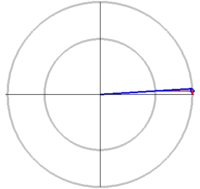Parametric equation for the ellipse (red) in canonical position. The eccentric anomaly t is the angle of the blue line with the X-axis.

For an ellipse in canonical position (center at origin, major axis along the X-axis), the equation simplifies to$X(t)=a\,\cos t$$Y(t)=b\,\sin t$

Note that the parameter t (called the eccentric anomaly in astronomy) is not the angle of (X(t),Y(t)) with the X-axis.

Formulae connecting a tangential angle ϕ, the angle anchored at the ellipse's center$\phi^\prime$ (called also the polar angle from the ellipse center), and the parametric angle t are:$\tan \phi=\frac {a}{b} \tan t=\frac {\tan \phi'}{(1-g)^2}=\frac {\tan \phi'}{1-e^2}$$\tan \phi^\prime=(1-f) \tan t$$\tan t=\frac {b}{a} \tan \phi=\sqrt{(1-e^2)} \tan \phi=(1-g) \tan \phi=\frac {\tan \phi'}{\sqrt{(1-e^2)}}=\frac {a}{b} \tan \phi'$

#### Polar form relative to center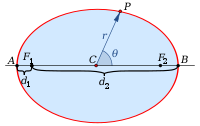Polar coordinates centered at the center.

In polar coordinates, with the origin at the center of the ellipse and with the angular coordinate θ measured from the major axis, the ellipse's equation is$r(\theta)=\frac{ab}{\sqrt{(b \cos \theta)^2 + (a\sin \theta)^2}}$

#### Polar form relative to focus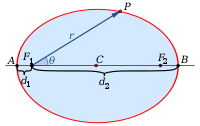Polar coordinates centered at focus.

If instead we use polar coordinates with the origin at one focus, with the angular coordinate θ = 0 still measured from the major axis, the ellipse's equation is$r(\theta)=\frac{a (1-e^{2})}{1 \pm e\cos\theta}$

where the sign in the denominator is negative if the reference direction θ = 0 points towards the center (as illustrated on the right), and positive if that direction points away from the center.

In the slightly more general case of an ellipse with one focus at the origin and the other focus at angular coordinate ϕ, the polar form is$r=\frac{a (1-e^{2})}{1 - e\cos(\theta - \phi)}.$

The angle θ in these formulas is called the true anomaly of the point. The numerator a(1 − e2) of these formulas is the semi-latus rectum of the ellipse, usually denoted l. It is the distance from a focus of the ellipse to the ellipse itself, measured along a line perpendicular to the major axis.Semi-latus rectum.

#### General polar form

The following equation on the polar coordinates (rθ) describes a general ellipse with semidiameters a and b, centered at a point (r0θ0), with the a axis rotated by φ relative to the polar axis:$r(\theta )=\frac{P(\theta )+Q(\theta )}{R(\theta )}$

where$P(\theta )=r_0 \left[\left(b^2-a^2\right) \cos \left(\theta +\theta _0-2 \varphi \right)+\left(a^2+b^2\right) \cos \left(\theta -\theta_0\right)\right]$$Q(\theta )=\sqrt{2} a b \sqrt{R(\theta )-2 r_0^2 \sin ^2\left(\theta -\theta_0\right)}$$R(\theta )=\left(b^2-a^2\right) \cos (2 \theta -2 \varphi )+a^2+b^2$

#### Angular eccentricity

The angular eccentricity α is the angle whose sine is the eccentricity e; that is,$\alpha=\sin^{-1}(e)=\cos^{-1}\left(\frac{b}{a}\right)=2\tan^{-1}\left(\sqrt{\frac{a-b}{a+b}}\right);\,\!$

### Degrees of freedom

An ellipse in the plane has five degrees of freedom (the same as a general conic section), defining its position, orientation, shape, and scale. In comparison, circles have only three degrees of freedom (position and scale), while parabolae have four. Said another way, the set of all ellipses in the plane, with any natural metric (such as the Hausdorff distance) is a five-dimensional manifold. These degrees can be identified with, for example, the coefficients A,B,C,D,E of the implicit equation, or with the coefficients Xc, Yc, φ, a, b of the general parametric form.

## Ellipses in physics

### Elliptical reflectors and acoustics

If the water's surface is disturbed at one focus of an elliptical water tank, the circular waves created by that disturbance, after being reflected by the walls, will converge simultaneously to a single point — the second focus. This is a consequence of the total travel length being the same along any wall-bouncing path between the two foci.

Similarly, if a light source is placed at one focus of an elliptic mirror, all light rays on the plane of the ellipse are reflected to the second focus. Since no other smooth curve has such a property, it can be used as an alternative definition of an ellipse. (In the special case of a circle with a source at its center all light would be reflected back to the center.) If the ellipse is rotated along its major axis to produce an ellipsoidal mirror (specifically, a prolate spheroid), this property will hold for all rays out of the source. Alternatively, a cylindrical mirror with elliptical cross-section can be used to focus light from a linear fluorescent lamp along a line of the paper; such mirrors are used in some document scanners.

Sound waves are reflected in a similar way, so in a large elliptical room a person standing at one focus can hear a person standing at the other focus remarkably well. The effect is even more evident under a vaulted roof shaped as a section of a prolate spheroid. Such a room is called a whisper chamber. The same effect can be demonstrated with two reflectors shaped like the end caps of such a spheroid, placed facing each other at the proper distance. Examples are the National Statuary Hall at the United States Capitol (where John Quincy Adams is said to have used this property for eavesdropping on political matters), at an exhibit on sound at the Museum of Science and Industry in Chicago, in front of the University of Illinois at Urbana-Champaign Foellinger Auditorium, and also at a side chamber of the Palace of Charles V, in the Alhambra.

### Planetary orbits

In the 17th century, Johannes Kepler discovered that the orbits along which the planets travel around the Sun are ellipses with the Sun at one focus, in his first law of planetary motion. Later, Isaac Newton explained this as a corollary of his law of universal gravitation.

More generally, in the gravitational two-body problem, if the two bodies are bound to each other (i.e., the total energy is negative), their orbits are similar ellipses with the common barycenter being one of the foci of each ellipse. The other focus of either ellipse has no known physical significance. Interestingly, the orbit of either body in the reference frame of the other is also an ellipse, with the other body at one focus.

Keplerian elliptical orbits are the result of any radially directed attraction force whose strength is inversely proportional to the square of the distance. Thus, in principle, the motion of two oppositely charged particles in empty space would also be an ellipse. (However, this conclusion ignores losses due to electromagnetic radiation and quantum effects which become significant when the particles are moving at high speed.)

For elliptical orbits, useful relations involving the eccentricity, e are:$e={{r_\mathrm{ap}-r_\mathrm{per}}\over{r_\mathrm{ap}+r_\mathrm{per}}}={{r_\mathrm{ap}-r_\mathrm{per}}\over{2a}}$$r_\mathrm{ap}=(1+e)a\!\,$$r_\mathrm{per}=(1-e)a\!\,$

where:

•$r_\mathrm{ap}\,\!$ is radius at apoapsis (i.e., the farthest distance).
•$r_\mathrm{per}\,\!$ is radius at periapsis (the closest distance).
•$a\,\!$ is the length of the semi-major axis.

In addition, the semimajor axis$a\,\!$ is the arithmetic mean of$r_\text{ap}\,\!$ and$r_\mathrm{per}\,\!$, or$a=\frac{{r_\text{ap}} + {r_\text{per}}}{2}$, and the semiminor axis$b\,\!$ is the geometric mean of$r_\text{ap}\,\!$ and$r_\mathrm{per}\,\!$, or$b=\, ^2 \! \! \! \! \sqrt{r_\mathrm{ap} \times r_\mathrm{per}}$ .
Also the semi-latus rectum (the distance from a focus to a point on the ellipse along a line parallel to the minor axis) is the harmonic mean of$r_\text{ap}\,\!$ and$r_\mathrm{per}\,\!$, or$\text {semi-latus rectum} = \frac{2}{\frac{1}{r_\text{ap}} + \frac{1}{r_\text{per}}}$ .

### Harmonic oscillators

The general solution for a harmonic oscillator in two or more dimensions is also an ellipse. Such is the case, for instance, of a long pendulum that is free to move in two dimensions; of a mass attached to a fixed point by a perfectly elastic spring; or of any object that moves under influence of an attractive force that is directly proportional to its distance from a fixed attractor. Unlike Keplerian orbits, however, these "harmonic orbits" have the center of attraction at the geometric center of the ellipse, and have fairly simple equations of motion.

### Phase visualization

In electronics, the relative phase of two sinusoidal signals can be compared by feeding them to the vertical and horizontal inputs of an oscilloscope. If the display is an ellipse, rather than a straight line, the two signals are out of phase.

### Elliptical gears

Two non-circular gears with the same elliptical outline, each pivoting around one focus and positioned at the proper angle, will turn smoothly while maintaining contact at all times. Alternatively, they can be connected by a link chain or timing belt, or in the case of a bicycle the main chainring may be elliptical, or an ovoid similar to an ellipse in form. Such elliptical gears may be used in mechanical equipment to produce variable angular speed or torque from a constant rotation of the driving axle, or in the case of a bicycle to allow a varying crank rotation speed with inversely varying mechanical advantage.

Elliptical bicycle gears make it easier for the chain to slide off the cog when changing gears.

An example gear application would be a device that winds thread onto a conical bobbin on a spinning machine. The bobbin would need to wind faster when the thread is near the apex than when it is near the base.

### Optics

In a material that is optically anisotropic (birefringent), the refractive index depends on the direction of the light. The dependency can be described by an index ellipsoid. (If the material is optically isotropic, this ellipsoid is a sphere.)

## Ellipses in statistics and finance

In statistics, a random vector (X, Y) is jointly elliptically distributed if its iso-density contours — loci of equal values of the density function — are ellipses. The concept extends to an arbitrary number of elements of the random vector, in which case in general the iso-density contours are ellipsoids. A special case is the multivariate normal distribution. The elliptical distributions are important in finance because if rates of return on assets are jointly elliptically distributed then all portfolios can be characterized completely by their mean and variance — that is, any two portfolios with identical mean and variance of portfolio return have identical distributions of portfolio return.

## Ellipses in computer graphics

Drawing an ellipse as a graphics primitive is common in standard display libraries, such as the MacIntosh QuickDraw API, and Direct2D on Windows. Jack Bresenham at IBM is most famous for the invention of 2D drawing primitives, including line and circle drawing, using only fast integer operations such as addition and branch on carry bit. M. L. V. Pitteway extended Bresenham's algorithm for lines to conics in 1967. Another efficient generalization to draw ellipses was invented in 1984 by Jerry Van Aken (IEEE CG&A, Sept. 1984).

In 1970 Danny Cohen presented at the "Computer Graphics 1970" conference in England a linear algorithm for drawing ellipses and circles. In 1971, L. B. Smith published similar algorithms for all conic sections and proved them to have good properties. These algorithms need only a few multiplications and additions to calculate each vector.

It is beneficial to use a parametric formulation in computer graphics because the density of points is greatest where there is the most curvature. Thus, the change in slope between each successive point is small, reducing the apparent "jaggedness" of the approximation.

### Drawing with Bezier spline paths

Multiple Bezier splines may also be used to draw an ellipse to sufficient accuracy, since any ellipse may be construed as an affine transformation of a circle. The spline methods used to draw a circle may be used to draw an ellipse, since the constituent Bezier curves will behave appropriately under such transformations.

## Line segment as a type of degenerate ellipse

A line segment is a degenerate ellipse with semi-minor axis = 0 and eccentricity = 1, and with the focal points at the ends. Although the eccentricity is 1 this is not a parabola. A radial elliptic trajectory is a non-trivial special case of an elliptic orbit, where the ellipse is a line segment.

## Ellipses in Optimization Theory

It is sometimes useful to find the minimum bounding ellipse on a set of points. The Ellipsoid method is quite useful for attacking this problem

Wikimedia Foundation. 2010.

Synonyms:

### Look at other dictionaries:

• Ellipse — geometrisch Die Saturnringe erscheinen elliptisch …   Deutsch Wikipedia

• Ellipse — (v. gr.), 1) Gramm.), Auslassung eines, zur Vollständigkeit eines Satzes nothwendigen, jedoch durch den Zusammenhang leicht zu ergänzenden Redetheiles. Die E. findet meist in, durch den Sprachgebrauch üblich gewordenen Redensarten, bes. in… …   Pierer's Universal-Lexikon

• ellipse — ELLIPSE. s. fém. Terme de Grammaire. Retranchement d un ou de plusieurs mots, qui seroient nécessaires pour la régularité de la construction, mais que l usage permet de supprimer. Quand on dit, la Saint Jean, pour dire, la fête de Saint Jean, c… …   Dictionnaire de l'Académie Française 1798

• Ellipse — El*lipse ([e^]l*l[i^]ps ), n. [Gr. e lleipsis, prop., a defect, the inclination of the ellipse to the base of the cone being in defect when compared with that of the side to the base: cf. F. ellipse. See {Ellipsis}.] 1. (Geom.) An oval or oblong… …   The Collaborative International Dictionary of English

• Ellipse — Sf ein Kegelschnitt ; Auslassung erw. fach. (17. Jh.) Entlehnung. Entlehnt aus l. ellīpsis, dieses aus gr. élleipsis, eigentlich Mangel, das Auslassen , einer Ableitung von gr. elleípein unterlassen, auslassen , zu gr. leípein lassen . Es wird… …   Etymologisches Wörterbuch der deutschen sprache

• Ellipse — Ellipse. Diejenige krumme Linie, welche man im gemeinen Leben mit dem Namen Eirund bezeichnet, wiewohl dieses falsch ist, weil das Eirund an einer Seite schmäler ist als an der andern, während die Ellipse an beiden Seiten auf völlig gleiche Weise …   Damen Conversations Lexikon

• ellipse — (n.) 1753, from Fr. ellipse (17c.), from L. ellipsis ellipse, also, a falling short, deficit, from Gk. elleipsis (see ELLIPSIS (Cf. ellipsis)). So called because the conic section of the cutting plane makes a smaller angle with the base than does …   Etymology dictionary

• Ellipse — (griech.), in der Grammatik Auslassung eines zur Vollständigkeit der Rede notwendigen, aber durch den grammatischen Zusammenhang leicht zu ergänzenden Satzteils, bildet sich leicht beim erregten Redner, wird aber auch in schriftlichen… …   Meyers Großes Konversations-Lexikon

• Ellipse — Ellipse: Zu griech. leípein »‹zurück›lassen«, das zur idg. Sippe von dt. ↑ leihen gehört, stellt sich die Bildung el leípein »darin zurücklassen; zurückstehen; mangeln, fehlen«. Das davon abgeleitete Substantiv griech. élleipsis »Mangel« wurde im …   Das Herkunftswörterbuch

• ellipse — [e lips′, ilips′] n. pl. ellipses [e lip′sēz΄, i lip′sēz΄] [ModL ellipsis < Gr elleipsis, a defect, ellipse < elleipein, to fall short < en , in + leipein, to leave (see LOAN): so named from falling short of a perfect circle] Geom. the… …   English World dictionary

• Ellipse — Ellipse, s. Kegelschnitte …   Lexikon der gesamten Technik